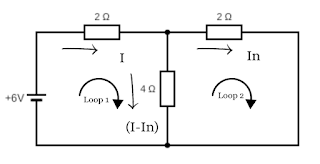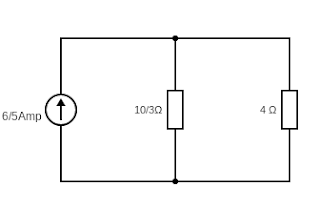# What is Norton's Theorem?

Norton’s theorem is just like Thevenin’s theorem. It is a theorem of transforming a complex linear circuit into a simple circuit. This theorem states that any two-terminal linear network or circuit containing energy sources and resistances can be replaced by an equivalent circuit containing a single current source IN in parallel with a resistance RN.

Where IN is the short circuit current (called Norton's current) at the terminals to which the load resistance is connected and RN is the resistance (called Norton's resistance) measured between the terminals with all energy sources are replaced by their internal resistances.

Here the simple circuit which is transformed from the complex circuit is known as Norton's equivalent circuit. However, there are some methods to find this Norton's equivalent circuit which we will know below. Before that let us know a little bit about the constant current source first.

What is a Constant Current source?

A constant current source delivers energy to a circuit such that the current drawn from the source is independent of the voltage across the terminals.

Let us consider a voltage source of emf E and internal resistance Ri supplying power to a load resistance RL.

The voltage at the terminals of the voltage source

If RL is varied, I remain constant although the terminal voltage Vt changes. Thus a voltage source behaves as a constant current source when its internal resistance is much larger than the load resistance. For an ideal current source, the internal resistance is infinite.

## Norton's equivalent circuit

To find Norton's equivalent circuit or simplifying any linear circuits we consider a circuit with a complicated linear network driven by an energy source (VS). Where the network also contains three resistors (R1, R2, and R3) and they are connected with a load (RL).

After Norton's conversion, this complicated linear network exactly looks like this...
Where the circuit contains just a single current source and parallel resistance connected to a load.

## Steps to remember to find out Norton’s Equivalent Circuit

• Identify the load resistance (RL).

• Replace the load with a short circuit and calculate the current (called Norton's current IN) through the short circuit.

• Replace the energy source with their internal resistances and calculate the resistance (called Norton's resistance RN) across the open ends.

## Problem no - 1: Calculate the current through the resistance 4Ω by applying Norton's Theorem.Solution:Identify the load resistance (RL):Here the load resistance is 4Ω.Find the Norton Current (IN):Now replace the load with a short circuit and calculate the current (called Norton's current IN) through the short circuit.By applying Kirchhoff's Voltage Law (KVL) in loop 1, we getBy applying Kirchhoff's Voltage Law (KVL) in loop 2, we getUsing equation no - (2) in equation no - (1), we getFind the Norton Resistance (RN):Now replace the energy source with their internal resistances and calculate the resistance (called Norton's resistance RN) across the open ends.Here,Norton's equivalent circuitWe connect the load resistance RL across the current source IN (Norton's current) shunted by the resistance RN.Now the voltage across the RN and RL in parallel is Therefore, the current through the load resistance RL is given byProblem no - 2: Calculate the current through the resistance RL = 5Ω by applying Norton's Theorem.Solution:Identify the load resistance (RL):Here the load resistance is 5Ω.Find the Norton Current (IN):Now replace the load with a short circuit and calculate the current (called Norton's current IN) through the short circuit.By applying Kirchhoff's Voltage Law (KVL) in loop 1, we get

By applying Kirchhoff's Voltage Law (KVL) in loop 2, we get

Using equation no - (2) in equation no - (1), we get

## Find the Norton Resistance (RN):Now replace the energy source with their internal resistances and calculate the resistance (called Norton's resistance RN) across the open ends.Here,

Norton's equivalent circuit

##Here RN and RL in parallel, so the equivalent resistance

Now the voltage across the RN and RL in parallel is

Therefore, the current through the load resistance RL is given by